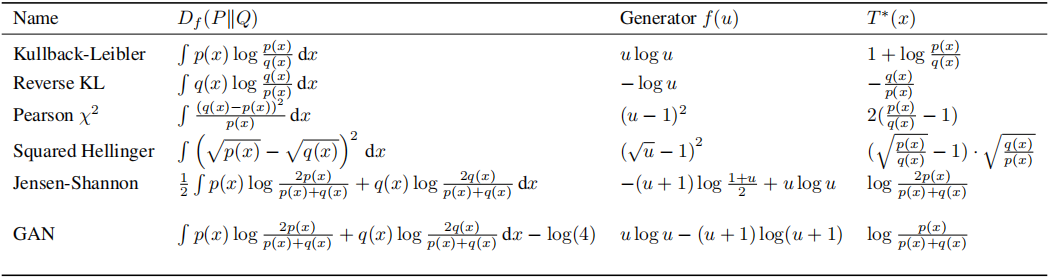# f-gan生成对抗神经网络进阶第一级

$D _ { f } ( P \| Q ) = \int _ { x } q ( x ) f \left( \frac { p ( x ) } { q ( x ) } \right) d x$

$D _ { f } ( P \| Q ) \geq f(\int _ { x } q ( x ) \left( \frac { p ( x ) } { q ( x ) } \right) d x)=f(1)=0$

$f ^ { * } ( t ) = \max _ { x \in \operatorname { dom } ( f ) } \{ x t - f ( x ) \}$

$f( x ) = \max _ { t \in \operatorname { dom } ( f ^ { * } ) } \{ x t - f ^ { * } ( t ) \}$

$D _ { f } ( P \| Q ) = \int _ { x } q ( x ) \max _ { t \in \operatorname { dom } ( f ^ { * } ) } \{ \frac { p ( x ) } { q ( x ) } t - f ^ { * } ( t ) \} d x$

$D _ { f } ( P \| Q ) = \int _ { x } q ( x ) \max _ { D(x) \in \operatorname { dom } ( f ^ { * } ) } \{ \frac { p ( x ) } { q ( x ) } D(x) - f ^ { * } ( D(x) ) \} d x$

$\geq\int _ { x } q ( x ) \{ \frac { p ( x ) } { q ( x ) } D(x) - f ^ { * } ( D(x) ) \} d x$

$=\int _ { x } p ( x )D(x) - q ( x )f ^ { * } ( D(x) ) d x$

$D_{f}(P \| Q) \approx \max _{\mathrm{D}} \{\int_{x} p(x) D(x) d x-\int_{x} q(x) f^{*}(D(x)) d x\}$

$=\max _{\mathrm{D}}\left\{E_{x \sim P}[D(x)]-E_{x \sim Q}\left[f^{*}(D(x))\right]\right\}$

$G^*=arg\min _{\mathrm{G}}\max _{\mathrm{D}}\left\{E_{x \sim P}[D(x)]-E_{x \sim Q}\left[f^{*}(D(G(x))\right]\right\}$### 手机扫码访问

•